# geoplot

Plot points, lines, and polygons on map

Since R2022a

## Syntax

``geoplot(GT)``
``geoplot(shape)``
``geoplot(___,LineSpec)``
``geoplot(lat,lon)``
``geoplot(lat,lon,LineSpec)``
``geoplot(lat1,lon1,...,latN,lonN)``
``geoplot(lat1,lon1,LineSpec1,...,latN,lonN,LineSpecN)``
``geoplot(tbl,latvar,lonvar)``
``geoplot(___,Name=Value)``
``geoplot(ax,___)``
``h = geoplot(___)``

## Description

Mapping Toolbox™ extends the functionality of the `geoplot` (MATLAB®) function. It adds support for displaying points, lines, and polygons with coordinates in any supported geographic or projected coordinate reference system (CRS). Depending on the type of axes, the function displays data into different map projections.

• Geographic axes — A Web Mercator projection

• Map axes — The projection specified by the `ProjectedCRS` property of the map axes

If you do not have Mapping Toolbox installed, then see `geoplot`.

### Geospatial Table and Shape Data

example

````geoplot(GT)` plots the point, line, or polygon shape objects within the `Shape` variable of the geospatial table `GT`. If the current axes is not a geographic or map axes, or if there is no current axes, then the function plots the line in a new geographic axes.```

example

````geoplot(shape)` plots the point, line, or polygon shape objects in `shape`.```

example

````geoplot(___,LineSpec)` sets the style, marker symbol, and color for the plotted shapes. You can use the `LineSpec` argument with either the `GT` or `shape` argument.```

### Numeric Vector Data

````geoplot(lat,lon)` plots a line with vertices at the latitudes and longitudes in `lat` and `lon`, respectively.```

example

````geoplot(lat,lon,LineSpec)` sets the style, marker symbol, and color for the line.```
````geoplot(lat1,lon1,...,latN,lonN)` plots multiple pairs of latitude and longitude coordinates on the same set of axes.```
````geoplot(lat1,lon1,LineSpec1,...,latN,lonN,LineSpecN)` assigns specific line styles, markers, and colors to each latitude-longitude pair.```

### Numeric Table Data

````geoplot(tbl,latvar,lonvar)` plots the variables `latvar` and `lonvar` from the table `tbl`. To plot one data set, specify one variable for `latvar` and one variable for `lonvar`. To plot multiple data sets, specify multiple variables for `latvar`, `lonvar`, or both. If both arguments specify multiple variables, they must specify the same number of variables. (Since R2022b)```

example

````geoplot(___,Name=Value)` specifies options for the plot using one or more name-value arguments, in addition to any combination of input arguments from the previous syntaxes.```

example

````geoplot(ax,___)` plots in the geographic axes or map axes specified by `ax`.```

example

````h = geoplot(___)` returns a `Point`, `Line`, or `Polygon` object. The type of object depends on the type of input. Use `h` to modify the properties of the plot object.```

## Examples

collapse all

Import a shapefile containing road data for Concord, MA, into the workspace as a geospatial table. The table represents roads using line shapes in projected coordinates.

```GT = readgeotable("concord_roads.shp"); GT.Shape```
```ans=609×1 object 16×1 maplineshape array with properties: NumParts: [16×1 double] Geometry: "line" CoordinateSystemType: "planar" ProjectedCRS: [1×1 projcrs] ⋮ ```

Display the line shapes by passing the table to the `geoplot` function.

```figure geoplot(GT)```

Change the basemap and add a title.

```geobasemap streets title("Road Network Over Streets Basemap")```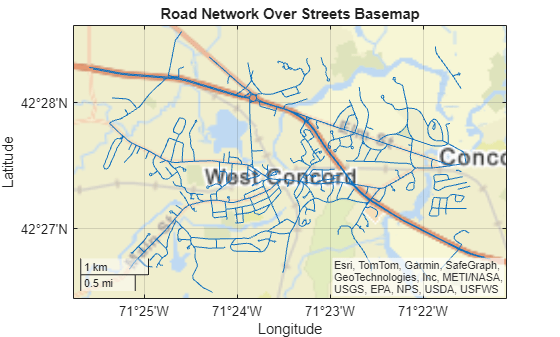One way to plot data from a geospatial table and customize the colors is to set the `ColorVariable` property. You can set this property by using a name-value argument when you call the `geoplot` function, or you can set it on the plot object later.

Read a shapefile containing tsunami events into the workspace as a geospatial table. The table represents the tsunami events using point shapes in geographic coordinates.

`GT = readgeotable("tsunamis.shp",CoordinateSystemType="geographic");`

Create a subtable containing events for a region surrounding Southeast Asia.

```bbox = geopolyshape([-25 35 35 -25 -25],[90 90 170 170 90]); inpoly = isinterior(bbox,GT.Shape); GT2 = GT(inpoly,:);```

Display the point shapes within the table. Vary the marker colors by specifying the `ColorVariable` name-value argument as a table variable. Return the `Point` object as `h`, so you can change the `ColorVariable` property later.

```figure h = geoplot(GT2,ColorVariable="Year",MarkerSize=20);```

```geobasemap grayterrain colorbar title("Tsunamis by Year")```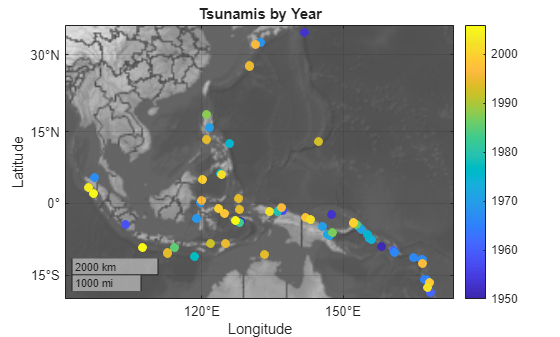Change the marker colors again by setting the `ColorVariable` property to a different table variable.

```h.ColorVariable = "Max_Height"; title("Tsunamis by Maximum Height")```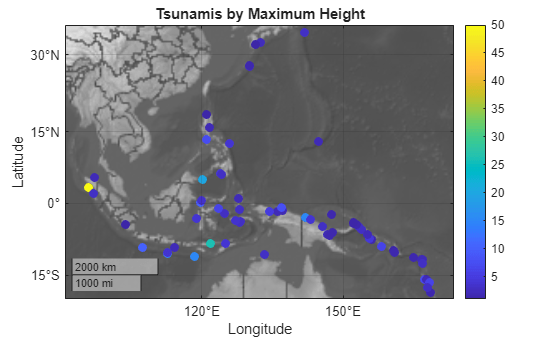Read a shapefile containing world cities into the workspace as a geospatial table. The table represents the cities using point shapes in geographic coordinates.

```GT = readgeotable("worldcities.shp"); shape = GT.Shape```
```shape = 318×1 geopointshape array with properties: NumPoints: [318×1 double] Latitude: [318×1 double] Longitude: [318×1 double] Geometry: "point" CoordinateSystemType: "geographic" GeographicCRS: [1×1 geocrs] ```

Clip the shapes to a region containing part of Europe.

`clipped = geoclip(shape,[30 60],[-20 35]);`

Display the shapes using red plus sign markers over a topographic basemap.

```figure geoplot(clipped,"r+") geobasemap topographic title("Cities Over Topographic Basemap")```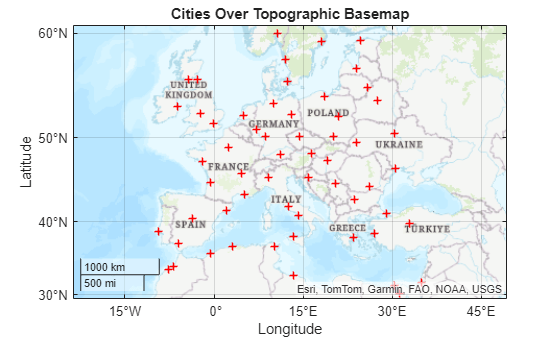Read hydrography data into the workspace as a geospatial table. The table represents the data using polygon shapes in projected coordinates. Extract the polygon shape for a pond.

```GT = readgeotable("concord_hydro_area.shp"); shape = GT.Shape(14)```
```shape = mappolyshape with properties: NumRegions: 1 NumHoles: 3 Geometry: "polygon" CoordinateSystemType: "planar" ProjectedCRS: [1x1 projcrs] ```

To plot shapes in projected coordinates using the `geoplot` function, the `ProjectedCRS` property of the shape must not be empty. View the contents of the `ProjectedCRS` property.

`shape.ProjectedCRS`
```ans = projcrs with properties: Name: "NAD83 / Massachusetts Mainland" GeographicCRS: [1x1 geocrs] ProjectionMethod: "Lambert Conic Conformal (2SP)" LengthUnit: "meter" ProjectionParameters: [1x1 map.crs.ProjectionParameters] ```

Create a new map that uses the same projected CRS as the pond polygon. Then, display the pond polygon.

```figure newmap(shape.ProjectedCRS) geoplot(shape)```

Title the map using the name of the projected CRS.

`title(shape.ProjectedCRS.Name)`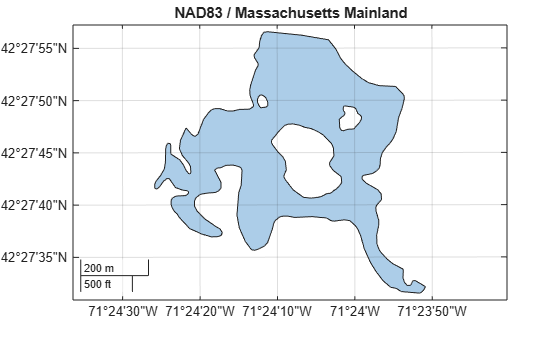Read a shapefile of US states into the workspace as a geospatial table. The table represents the states using polygon shapes in geographic coordinates.

```GT = readgeotable("usastatehi.shp"); shape = GT.Shape;```

Clip the shapes to a region containing the conterminous US.

`clipped = geoclip(shape,[17 56],[-127 -65]);`

Create a new map that uses a North America Albers Equal Area Conic projection. Then, display the shapes. Vary the colors by using the `ColorData` name-value argument.

```figure proj = projcrs(102008,Authority="ESRI"); newmap(proj) c = 1:length(clipped); geoplot(clipped,ColorData=c)```

`title("Conterminous US")`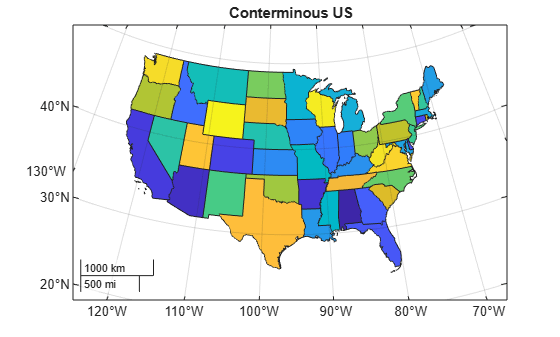Load a MAT file containing the coordinates of global coastlines into the workspace. The variables within the MAT file, `coastlat` and `coastlon`, specify numeric latitude and longitude coordinates, respectively. Display the coordinates using a blue line over a topographic basemap.

```load coastlines figure geoplot(coastlat,coastlon,"b") geobasemap topographic```

Read the geographic coordinates of European capitals into the workspace. Display the capitals using magenta circle markers on the same map.

```[lat,lon] = readvars("european_capitals.txt"); hold on geoplot(lat,lon,"om",MarkerFaceColor="m") title("European Capitals Over Topographic Basemap")```

Center the map over Europe by changing its limits.

`geolimits([30 60],[-13 43])`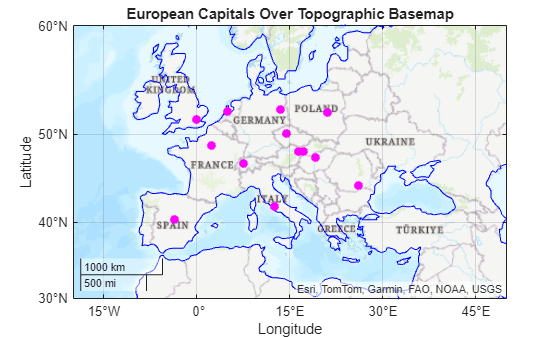Import several shapefiles into the workspace as geospatial tables.

• `landareas.shp` contains world land areas. The table represents the areas using polygon shapes in geographic coordinates (`geopolyshape` objects).

• `worldrivers.shp` contains world rivers. The table represents the rivers using line shapes in geographic coordinates (`geolineshape` objects).

• `worldcities.shp` contains world cities. The table represents the cities using point shapes in geographic coordinates (`geopointshape` objects).

```land = readgeotable("landareas.shp"); rivers = readgeotable("worldrivers.shp"); cities = readgeotable("worldcities.shp");```

Set up a new map. By default, map axes use an Equal Earth map projection. Then, display each set of shapes using separate calls to the `geoplot` function. Different shapes support different name-value arguments.

• Display the land areas using green polygons.

• Display the rivers using blue lines.

• Display the cities using black points.

```figure newmap hold on geoplot(land,FaceColor=[0.7 0.9 0.5],EdgeColor=[0.7 0.9 0.5]) geoplot(rivers,Color=[0 0.4470 0.7410]) geoplot(cities,"k")```

`title("World Land Areas, Rivers, and Cities")`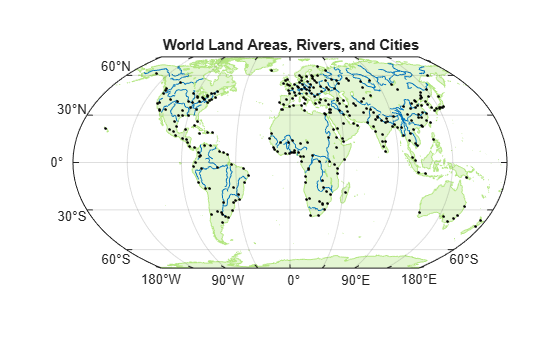Create multiple geographic axes in a single figure by using a tiled chart layout.

Create a 1-by-2 tiled chart layout.

`t = tiledlayout(1,2);`

Load a MAT file containing the coordinates of global coastlines into the workspace. The variables within the MAT file, `coastlat` and `coastlon`, specify numeric latitude and longitude coordinates, respectively.

`load coastlines`

Place a geographic axes in the first tile. Plot the coordinates as a line on the axes.

```gx1 = geoaxes(t); geoplot(gx1,coastlat,coastlon) title(gx1,"Coastline Coordinates")```

Read a shapefile containing world land areas into the workspace as a geospatial table. The table represents the land areas using polygon shapes in geographic coordinates.

`GT = readgeotable("landareas.shp");`

Place a new geographic axes in the second tile. Plot the land areas as a polygon on the new axes.

```gx2 = geoaxes(t); gx2.Layout.Tile = 2; geoplot(gx2,GT) title(gx2,"Land Areas")```

Zoom both axes to a region containing Africa.

```latlim = [-55 60]; lonlim = [-21 53]; geolimits(gx1,latlim,lonlim) geolimits(gx2,latlim,lonlim)```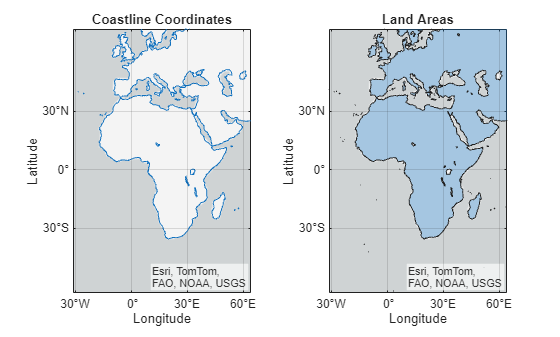Import several shapefiles into the workspace as geospatial tables.

• `landareas.shp` contains world land areas. The table represents the areas using polygon shapes in geographic coordinates (`geopolyshape` objects).

• `worldrivers.shp` contains world rivers. The table represents the rivers using line shapes in geographic coordinates (`geolineshape` objects).

• `worldcities.shp` contains world cities. The table represents the cities using point shapes in geographic coordinates (`geopointshape` objects).

```land = readgeotable("landareas.shp"); rivers = readgeotable("worldrivers.shp"); cities = readgeotable("worldcities.shp");```

Display each set of shapes by using separate calls to the `geoplot` function.

• Display the land areas and return the polygon in `h1`. The polygon in `h1` represents multiple polygon shapes in `land`.

• Display the rivers and return the line in `h2`. The line in `h2` represents multiple line shapes in `rivers`.

• Display the cities and return the point in `h3`. The point in `h3` represents multiple point shapes in `cities`.

```figure h1 = geoplot(land); hold on h2 = geoplot(rivers); h3 = geoplot(cities);```

Change the geographic limits of the map, add a title, and remove the basemap.

```geolimits([-72 85],[-180 180]) title("World Land Areas, Rivers, and Cities") geobasemap none```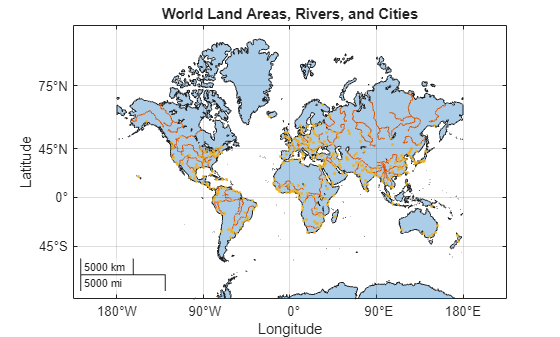Update properties of the polygon, point, and line objects. Each object supports different properties.

• Change the fill and outline colors of the polygons to green.

• Change the color of the lines to blue.

• Change the color of the markers to black.

```h1.FaceColor = [0.7 0.9 0.5]; h1.EdgeColor = [0.7 0.9 0.5]; h2.Color = [0 0.4470 0.7410]; h3.MarkerEdgeColor = "k";```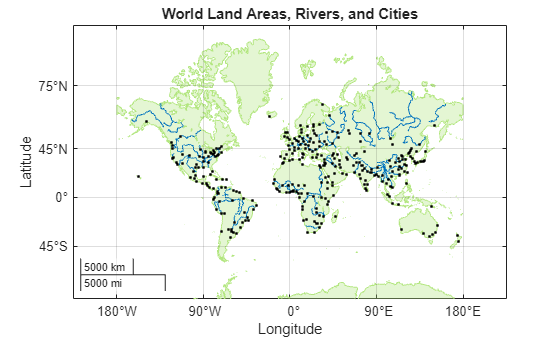## Input Arguments

collapse all

Geospatial table. A geospatial table is a `table` or `timetable` object with a `Shape` variable that contains point, line, or polygon shapes. For more information about geospatial tables, see Create Geospatial Tables.

The `Shape` variable of the table must contain only one type of shape.

The `ProjectedCRS` property of `mappointshape`, `maplineshape`, and `mappolyshape` objects within the `Shape` variable must not be empty.

If the `GeographicCRS` property of a `geopointshape`, `geolineshape`, or `geopolyshape` object within the `Shape` variable is empty, then the function assumes the geographic CRS based on the type of axes:

• Geographic axes — The WGS84 coordinate reference system.

• Map axes — The geographic CRS specified by the `ProjectedCRS` property of the map axes. To find the geographic CRS, access the projected CRS in the `ProjectedCRS` property. Then, access the `GeographicCRS` property of the projected CRS. For example, to find the geographic CRS for a map axes `mx`, query `mx.ProjectedCRS.GeographicCRS`.

Point, line, or polygon shapes, specified as one of these options:

You can also specify this argument as a scalar point, line, or polygon shape.

The `ProjectedCRS` property of `mappointshape`, `maplineshape`, and `mappolyshape` objects must not be empty.

If the `GeographicCRS` property of a `geopointshape`, `geolineshape`, or `geopolyshape` object within the `Shape` variable is empty, then the function assumes the geographic CRS based on the type of axes:

• Geographic axes — The WGS84 coordinate reference system.

• Map axes — The geographic CRS specified by the `ProjectedCRS` property of the map axes. To find the geographic CRS, access the projected CRS in the `ProjectedCRS` property. Then, access the `GeographicCRS` property of the projected CRS. For example, to find the geographic CRS for a map axes `mx`, query `mx.ProjectedCRS.GeographicCRS`.

Latitude coordinates in degrees, specified as a vector. The vector can contain `NaN` values.

Depending on the type of axes, the `geoplot` function references numeric coordinates to different geographic CRSs.

• Geographic axes — The WGS84 coordinate reference system. To plot points or lines with coordinates in a different CRS, use the coordinates to create a `geopointshape` or `geolineshape` object and set its `GeographicCRS` property. Then, pass the object you created to the `geoplot` function.

• Map axes — The geographic CRS specified by the `ProjectedCRS` property of the map axes. To find the geographic CRS, access the projected CRS in the `ProjectedCRS` property. Then, access the `GeographicCRS` property of the projected CRS. For example, to find the geographic CRS for a map axes `mx`, query `mx.ProjectedCRS.GeographicCRS`.

`lat` must be the same size as `lon`.

Example: `[43.0327 38.8921 44.0435]`

Data Types: `single` | `double`

Longitude coordinates in degrees, specified as a vector. The vector can contain `NaN` values.

Depending on the type of axes, the `geoplot` function references numeric coordinates to different geographic CRSs.

• Geographic axes — The WGS84 coordinate reference system. To plot points or lines with coordinates in a different CRS, use the coordinates to create a `geopointshape` or `geolineshape` object and set its `GeographicCRS` property. Then, pass the object you created to the `geoplot` function.

• Map axes — The geographic CRS specified by the `ProjectedCRS` property of the map axes. To find the geographic CRS, access the projected CRS in the `ProjectedCRS` property. Then, access the `GeographicCRS` property of the projected CRS. For example, to find the geographic CRS for a map axes `mx`, query `mx.ProjectedCRS.GeographicCRS`.

`lon` must be the same size as `lat`.

Example: `[-107.5556 -77.0269 -72.5565]`

Data Types: `single` | `double`

Line style, marker, and color, specified as a character vector or string scalar containing symbols. You can specify the symbols in any order. Different types of input support different characteristics (line, marker style, and color).

Type of InputSupported CharacteristicsExample
`GT` or `shape` contains `geopointshape` or `mappointshape` objects

Marker and color

`'ro'` specifies red circle markers
`GT` or `shape` contains `geolineshape` or `maplineshape` objects

Line style and color

`'r--'` specifies red dashed lines
`GT` or `shape` contains `geopolyshape` or `mappolyshape` objects

Line style and color

`'r--'` specifies red dashed lines
`lat` and `lon` contain numeric data

Line style, marker, and color

`'--or'` specifies red dashed lines with circle markers

You do not need to specify all supported characteristics. For example, if you plot a line from numeric data and specify only the marker, then the plot shows only the marker and no line.

Line StyleDescriptionResulting Line
`"-"`Solid line`"--"`Dashed line`":"`Dotted line`"-."`Dash-dotted lineMarkerDescriptionResulting Marker
`"o"`Circle`"+"`Plus sign`"*"`Asterisk`"."`Point`"x"`Cross`"_"`Horizontal line`"|"`Vertical line`"square"`Square`"diamond"`Diamond`"^"`Upward-pointing triangle`"v"`Downward-pointing triangle`">"`Right-pointing triangle`"<"`Left-pointing triangle`"pentagram"`Pentagram`"hexagram"`HexagramColor NameShort NameRGB TripletAppearance
`"red"``"r"``[1 0 0]``"green"``"g"``[0 1 0]``"blue"``"b"``[0 0 1]``"cyan"` `"c"``[0 1 1]``"magenta"``"m"``[1 0 1]``"yellow"``"y"``[1 1 0]``"black"``"k"``[0 0 0]``"white"``"w"``[1 1 1]`Source table containing the data to plot, specified as a table or a timetable.

Table variables containing the latitude coordinates, specified using one of the indexing schemes from the table.

Indexing SchemeExamples

Variable names:

• `"A"` or `'A'` — A variable called `A`

• `["A","B"]` or `{'A','B'}` — Two variables called `A` and `B`

• `"Var"+digitsPattern(1)` — Variables named `"Var"` followed by a single digit

Variable index:

• An index number that refers to the location of a variable in the table.

• A vector of numbers.

• A logical vector. Typically, this vector is the same length as the number of variables, but you can omit trailing `0` or `false` values.

• `3` — The third variable from the table

• `[2 3]` — The second and third variables from the table

• `[false false true]` — The third variable

Variable type:

• `vartype("categorical")` — All the variables containing categorical values

Regardless of the variable name, the axis label on the plot is always `Latitude`.

The variables you specify must contain numeric data of type `single` or `double`. The data must be in the range (–90, 90).

If `latvar` and `lonvar` both specify multiple variables, the number of variables must be the same.

Example: `geoplot(tbl,["lat1","lat2"],"lon")` specifies the table variables named `lat1` and `lat2` for the latitude coordinates.

Example: `geoplot(tbl,2,"lon")` specifies the second variable for the latitude coordinates.

Example: `geoplot(tbl,vartype("numeric"),"lon")` specifies all numeric variables for the latitude coordinates.

Table variables containing the longitude coordinates, specified using one of the indexing schemes from the table.

Indexing SchemeExamples

Variable names:

• `"A"` or `'A'` — A variable called `A`

• `["A","B"]` or `{'A','B'}` — Two variables called `A` and `B`

• `"Var"+digitsPattern(1)` — Variables named `"Var"` followed by a single digit

Variable index:

• An index number that refers to the location of a variable in the table.

• A vector of numbers.

• A logical vector. Typically, this vector is the same length as the number of variables, but you can omit trailing `0` or `false` values.

• `3` — The third variable from the table

• `[2 3]` — The second and third variables from the table

• `[false false true]` — The third variable

Variable type:

• `vartype("categorical")` — All the variables containing categorical values

Regardless of the variable name, the axis label on the plot is always `Longitude`.

The variables you specify must contain numeric data of type `single` or `double`.

If `latvar` and `lonvar` both specify multiple variables, the number of variables must be the same.

Example: `geoplot(tbl,"lat",["lon1","lon2"])` specifies the table variables named `lon1` and `lon2` for the longitude coordinates.

Example: `geoplot(tbl,"lat",2)` specifies the second variable for the longitude coordinates.

Example: `geoplot(tbl,"lat",vartype("numeric"))` specifies all numeric variables for the longitude coordinates.

Target axes, specified as a `GeographicAxes` object1 or `MapAxes` object. If you do not specify this argument, then the `geoplot` function plots into the current axes, provided that the current axes is a geographic or map axes object.

### Name-Value Arguments

Specify optional pairs of arguments as `Name1=Value1,...,NameN=ValueN`, where `Name` is the argument name and `Value` is the corresponding value. Name-value arguments must appear after other arguments, but the order of the pairs does not matter.

Example: `geoplot(47,-122,Marker="*",Color="m")` displays a magenta star marker at the specified latitude and longitude.

Specify properties for the plot by using name-value arguments. The supported name-value arguments depend on the type of input.

Type of Input Supported Name-Value Arguments
`GT` or `shape` contains `geopointshape` or `mappointshape` objectsSee Point Properties
`GT` or `shape` contains `geolineshape` or `maplineshape` objectsSee Line Properties
`GT` or `shape` contains `geopolyshape` or `mappolyshape` objectsSee Polygon Properties
`lat` and `lon` contain numeric dataSee Line Properties

## Output Arguments

collapse all

Plot object. The value of `h` depends on the type of input you pass to the `geoplot` function.

Type of Input Value of `h`
`GT` or `shape` contains `geopointshape` or `mappointshape` objectsA geographic `Point` object. A `Point` object can represent multiple `geopointshape` or `mappointshape` objects.
`GT` or `shape` contains `geolineshape` or `maplineshape` objectsA geographic chart `Line` object. A `Line` object can represent multiple `geolineshape` or `maplineshape` objects.
`GT` or `shape` contains `geopolyshape` or `mappolyshape` objectsA geographic `Polygon` object. A `Polygon` object can represent multiple `geopolyshape` or `mappolyshape` objects.
`lat` and `lon` contain numeric data

A column vector of chart `Line` objects. Each object corresponds to a vector of latitude-longitude pairs.

## Version History

Introduced in R2022a

expand all